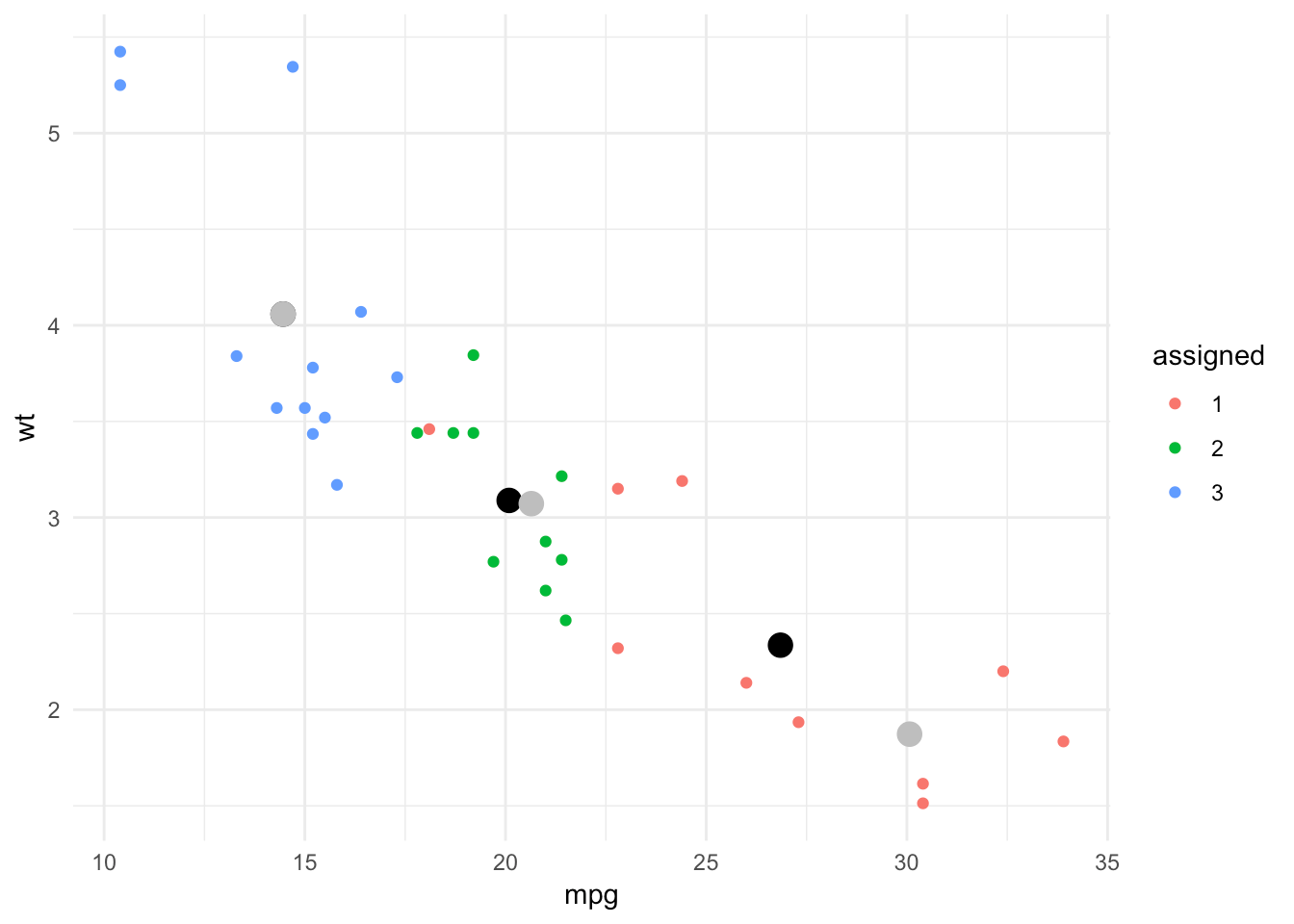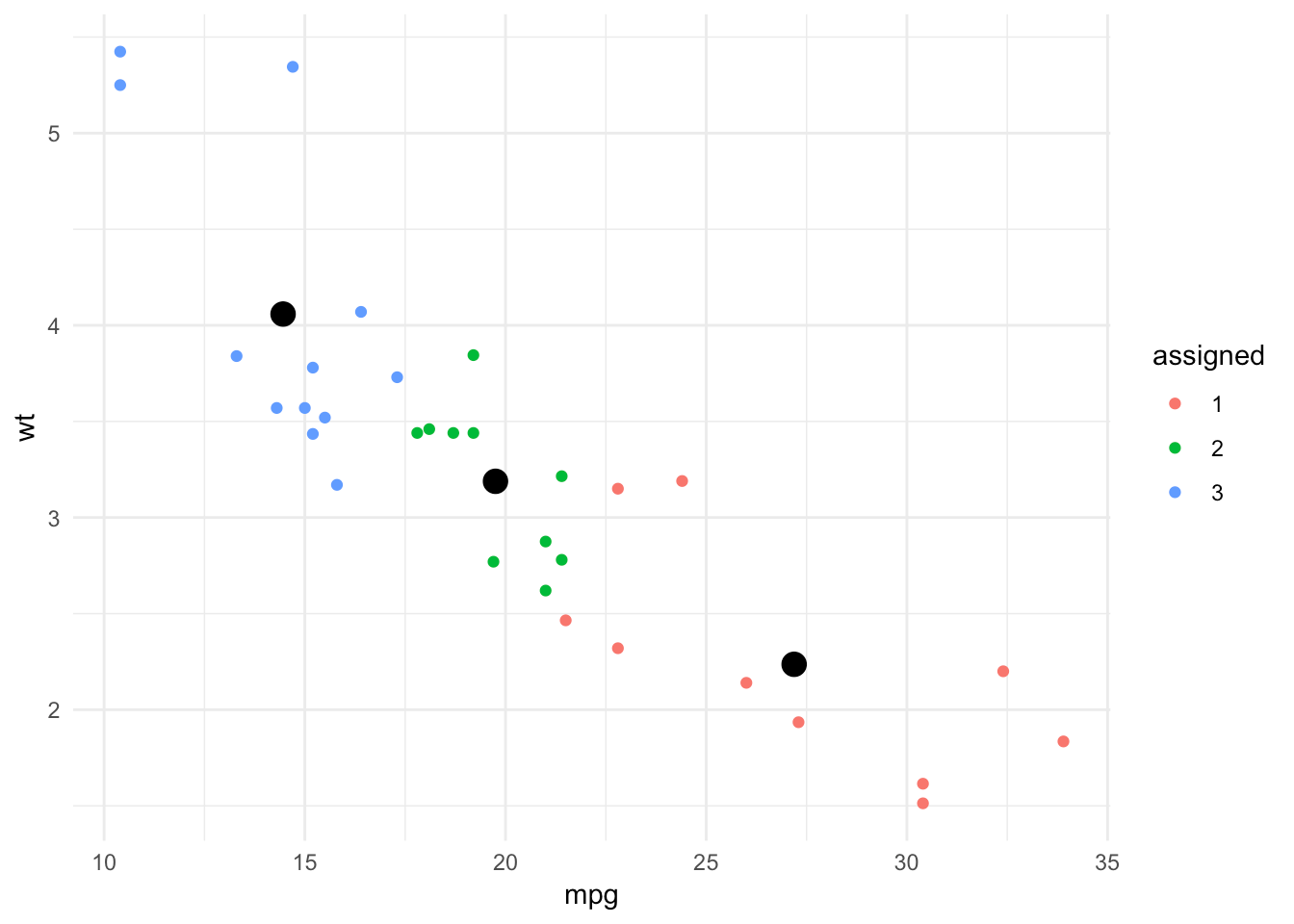Want to share your content on R-bloggers? click here if you have a blog, or here if you don't.

We were recently presented with a problem where the decision maker wanted to understand how their data would naturally group together. The classic technique of k-means clustering was a natural choice; it’s well known, computationally efficient, and implemented in base R via the `kmeans()` function.

Our problem has a slight wrinkle: the decision maker wished to see the data grouped with (nearly) equal sizes. Now, a ‘true’ statistician would tell the client that the right thing to do from a theoretical perspective was to use native k-means results because some centers can simply have more nearby points than other centers. However, we are practitioners, and if the visualization provides additional information useful to the way people make decisions, we are not going to tell them they are wrong!

## Approach

This is very similar to a mathematical optimization problem commonly faced by organizations like fire and police departments; specifically, ‘where trucks/patrol cars should be stationed to minimize response time’.

The general strategy is to decompose the hard problem into two easier sub-problems, to wit:

1. If we knew where the centroids were, determining group membership would be easy.
2. If we knew group membership, determining centroids would be trivial.

The key insight (and it really is all downhill from here) is to simply pretend that we have the solution to issue 1, and iterate between these two tasks until convergence is reached, that is – make a guess at where the centroids are, pick group members, then adjust the centroid based on group membership. This has the same ‘feel’ as mathematical induction, and we’ll name the steps accordingly.

Our example is based on `mtcars` a built-in R dataset, with three clusters of equal size.

First, some libraries:

`library(magrittr); library(dplyr); library(ggplot2)`

### Basis Step

We have to start somewhere, and in this example, we will use an initial solution coming from the basic `kmeans` algorithm. Another approach would be to pick initial centroids at the ‘corners’ of the space, or to simply pick a few random data points as centroids:

```data(mtcars)
k = 3
kdat = mtcars %>% select(c(mpg, wt))
kdat %>% kmeans(k) -> kclust```

So far, so good. Now we’ll compute the distance matrix between each point and each centroid; this begins the

### Assignment step

```kdist = function(x1, y1, x2, y2){
sqrt((x1-x2)^2 + (y1-y2)^2)
}
centers = kclust\$centers

kdat %<>%
mutate(D1 = kdist(mpg, wt, centers[1,1], centers[1,2]))
kdat %<>%
mutate(D2 = kdist(mpg, wt, centers[2,1], centers[2,2]))
kdat %<>%
mutate(D3 = kdist(mpg, wt, centers[3,1], centers[3,2]))```

From here, we assign clusters, which we do greedily, using a technique we like to call ‘little kids soccer’ – because this is the way kids generally pick teams – by going in order and picking the ‘best’ option available to them at the time. The algorithm interrogates each cluster in turn and picks the ‘closest’ unassigned member until each cluster is filled. There’s one minor wrinkle that needed to be worked out: the final round consists of the ones that are the ‘worst fits’ across all k clusters; in this case, the points choose the clusters.

```kdat\$assigned = 0
kdat\$index = 1:nrow(kdat)
working = kdat
FirstRound = nrow(kdat) - (nrow(kdat) %% k)

for(i in 1:FirstRound){
#cluster counts can be off by 1 due to uneven multiples of k.
j = if(i %% k == 0) k else (i %% k)
itemloc =
working\$index[which(working[,(paste0("D", j))] ==
min(working[,(paste0("D",j))]))]
kdat\$assigned[kdat\$index == itemloc] = j
working %<>% filter(!index == itemloc)
##The sorting hat says... GRYFFINDOR!!!
}
for(i in 1:nrow(working)){
#these leftover points get assigned to whoever's closest, without regard to k
kdat\$assigned[kdat\$index ==
working\$index[i]] =
which(working[i,3:5] == min(working[i, 3:5]))
}```

Next, we recalculate the centroids. It’s kind of smooth to simply use `k-means` with `k = 1`.

```NewCenters <- kdat %>% filter(assigned == 1) %>%
select(mpg, wt) %>%
kmeans(1) %\$% centers

NewCenters %<>% rbind(kdat %>%
filter(assigned == 2) %>%
select(mpg, wt) %>%
kmeans(1) %\$% centers)

NewCenters %<>% rbind(kdat %>%
filter(assigned == 3) %>%
select(mpg, wt) %>%
kmeans(1) %\$% centers)

NewCenters %<>% as.data.frame()```

The result a single round is presented here:

```kdat\$assigned %<>% as.factor()
kdat %>% ggplot(aes(x = mpg, y = wt, color = assigned)) +
theme_minimal() + geom_point() +
geom_point(data = NewCenters, aes(x = mpg, y = wt),
color = "black", size = 4) +
geom_point(data = as.data.frame(centers),
aes(x = mpg, y = wt), color = "grey", size = 4)```Figure 1: Iterated k-means with one step

You will notice there is a single point assigned to Group 1 that is on the ‘frontier’ between Groups 2 and 3. This point appears to be misclassified, and the way to resolve this is to iterate the algorithm (see below).

You can see how coercing the size made the cluster centroids migrate – significantly in the case of the higher mpg cluster. Grey dots are the original centroid, black are the updated (equal size) centroid.

### Functionalized and iterated

It is straightforward to ‘wrap’ the above code into a function (truncated here for brevity), which we call `kMeanAdj`. It and takes the incumbent centers, data, number of iterations, and \(k\) as arguments. We may plot the result as follows:

```x = kMeanAdj(NewCenters, kdat, iter = 3, k)

x\$Data\$assigned %<>% as.factor()

x\$Data %>% ggplot(aes(x = mpg, y = wt, color = assigned)) +
theme_minimal() +  geom_point() +
geom_point(data = x\$centers, aes(x = mpg, y=wt),
color = "black", size = 4)```Figure 2: Equal Size Clusters with 3 iterations

Iterating the algorithm over several steps ‘stabilizes’ both the groups and centers, yielding the desired characteristics.

### Summary

Programming projects like this can sometimes feel like traveling by hot air balloon, in the sense that you don’t know which way you will be headed until you begin to travel. In this case, we did not initially anticipate the poor performance of our initial method in the case where `k` does not divide `n`. The only way to discover issues like this, of course, is to frequently prototype and test code. Overcoming this challenge added both to the fun and the reward of this exercise. Additionally, it showcases how the (robust) existing routines in the R language and popular packages may be rapidly combined with new ideas. This flexibility is what makes R a natural choice for both practitioners and theorists in Statistics and Operations Research.

Harrison Schramm, CAP, PStat, is a Senior Fellow at the Center for Strategic and Budgetary Assessment.

Carol DeZwarte, CAP, PMP, whose passion for advanced analytics predates it becoming a buzzword, is in Supply Chain Analytics at Wayfair.